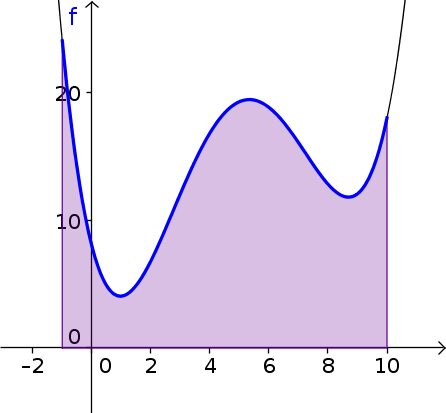# Math Insight

### Image: Shaded area under a curveThe area underneath a curve, i.e., the graph of a function, and above an interval on the x-axis is illustrated as a shaded region. The function is $f(x)=\frac{x^4}{20} -(x-2)^3+3x$ and the interval is $[-1, 10]$.